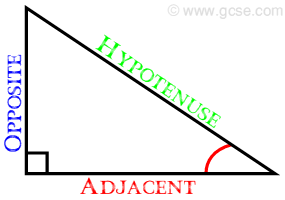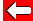# Pythagoras's Theorem

One ancienct Greek mathematician and philosopher, Pythagoras (c. 580 - 500 BC) determined a truly remarkable relationship between the lengths of the sides of right-angle triangles:hypotenuse² = opposite² + adjacent²

This is known as Pythagoras's Theorem and it works for all right-angled triangles. Let's see it in action.Go back a pageMaths MenuGo to next page